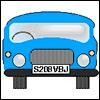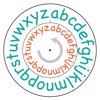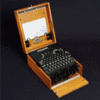#### You may also like### Numbered Cars

I was looking at the number plate of a car parked outside. Using my special code S208VBJ adds to 65. Can you crack my code and use it to find out what both of these number plates add up to?### Some Secret Codes

Can you follow the rule to decode the messages?### James's Code Challenge

The Enigma Project's James Grime has created a video code challenge. Watch it here!

# Unlocking the Case

##### Age 7 to 11Challenge Level

Well it has been very interesting looking at over $80$ solutions that came in with over $20$ different ideas as to what could unlock the case. Here are the codes and the names of those who suggested them.

$1916$ because T is the $19$th letter from Lance

$1652$ because T$16$ must be a sorting code from Rumanpreet

$916$ because T means Total from Tommy

$816$ because T is at the $8$ on a phone from Danny, Tubby, Daniel, Connor, Josh, Evie, Maddy and Portia

$773$ because T$16$ means $17$ so it has to add to that from Ediz

$716$ because T is $7$th from the end of the alphabet from Class 4/5B, Koya, Drew and Jje.

$672$ from Paneet, Any and Soneeshun for no particular reason

$376$ Yriel suggested this by using formula

$320$ because T is the $20$th letter and $20$x$16$ from Mattias, Georgia, Evie, Callum and Angus

$316$ because T means $10$ and they have to add to ten from Molescroft Primary School

$256$ because T means Times from William, Harry

$216$ because T is the $20$th and there's only $3$ spaces from Ben, Rowan,Kavo, Evie, Maddy, Portia, Nika and Ryan, Jake

$207$ because T is the $20$th and $1+6=7$ from Luke M, Danyal and Zak

$160$ because T means Tens from 'Primary 5', Asher, Sarah, Saloa, Michael, Hiruthika, Kieran and Ethan, another Ethan

$136$ this was the most popular answer with pupils considering that the T meant triangular number. Those who suggested this were Molly, Harry, Josie, 'Best Friends', Tallulah, 'ICT(2-)' 'GROUP1', Miles, Anna, George, Ari and Shail, Surichi, James

$116$ because T means Tens and you loose the Zero from Alya, Tom and Isaac. Isaiah also went for this number pointing out that T is $1$ in Scrabble, also because T means Twice, two $1$'s and then put the $6$ from Noor

T$48$ because T means $3$ times from Zamira

$048$ because T means $3$ times from Miles and Kieran

$20$P becuase T is the $20$th and the $16$th letter is P from Charlotte and Laura

Now look at what Sophia wrote;

You go to the Collins Cobuild Student Dictionary and find the T section you, find the page number, $713$ add on the $16$, use the initials of the dictionaries names number $3, 3, 19, 4,$ add them all together and you get $761$

A special mention to all those from Salcombe Prep School who sent in separate solutions, namely, Tallulah, Callim, Alya, Danyal. Surichi, Zamira, Anna, George, Ari, Nika, Charlie, Louis and Ediz.

Well done all of you, there are some really good and creative ideas to this challenge. Keep up your good mathematics work!